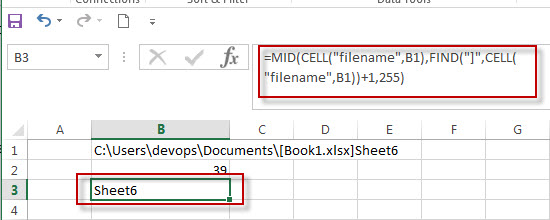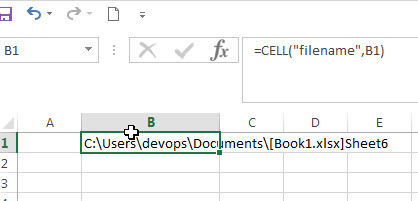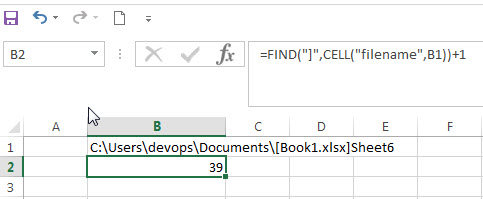# Get the Current Worksheet Name only

If you want to get the current worksheet name only in excel, you can use a combination of the MID function, the CELL function and FIND function. You can use the below generic formula:

`=MID(CELL("filename",B1),FIND("]",CELL("filename",B1))+1,255)`The below explains how the above excel formula works:

The CELL function will get the full information of the current worksheet contain the full file name and its path.

=CELL(“filename”,B1)The FIND function will locate the position of the right bracket symbol in the result returned by CELL function.

So the starting position of the filename of the current workbook can be got from the below formula:

`=FIND("]",CELL("filename",B1))+1`The MID function will extract the worksheet name from a text string returned by CELL function at the position that returned by the FIND function.